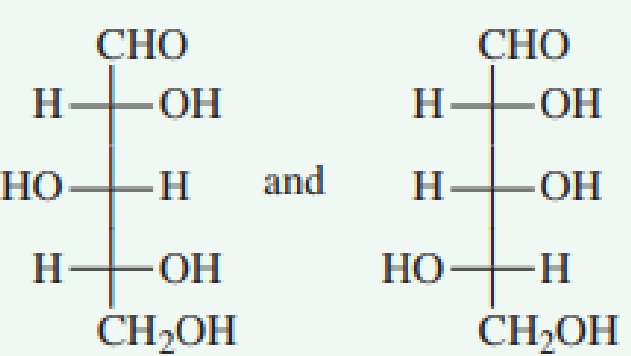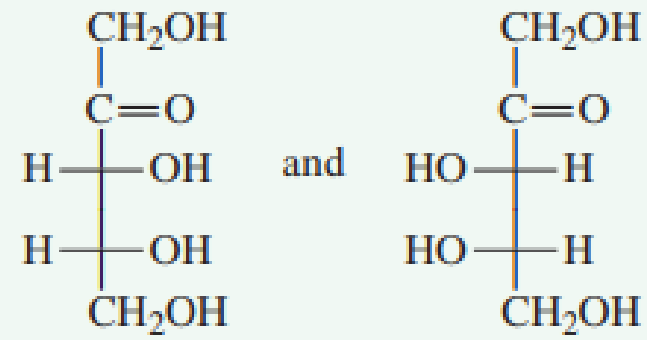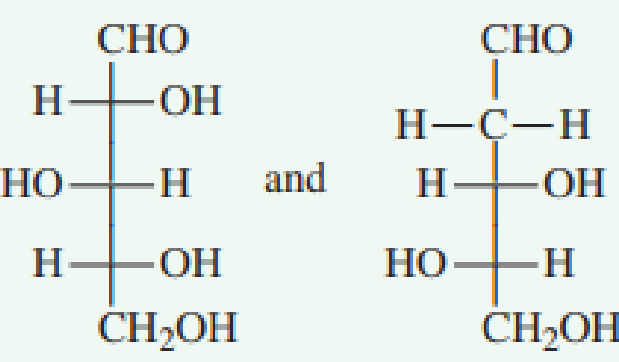Chapter 7.6, Problem 3QQ### Organic And Biological Chemistry

7th Edition
STOKER + 1 other
ISBN: 9781305081079

#### Solutions

Chapter
Section### Organic And Biological Chemistry

7th Edition
STOKER + 1 other
ISBN: 9781305081079
Textbook Problem

# In which pair of Fischer projection formulas are the two monosaccharides enantiomers?a. no correct response

Interpretation Introduction

Interpretation: The pair of Fisher projection formulas which represents two monosaccharides enantiomers has to be predicted.

Concept introduction: The two dimensional structural notation which represents the spatial arrangement of group around the chiral centers in molecules is known as the Fisher projection formula.  The chiral centre is indicated by the intersection of horizontal and vertical lines.  The two isomers which exhibit a mirror-image relationship at each chiral center are known as enantiomers.  The stereoisomers which are not mirror images of each other are known as diastereomers.

Explanation

Reason for correct option:

The pair of Fisher projection formulas in option (b) is shown below.

In the first Fisher projection, both chiral center OH groups are present on the right side.  In the second Fisher projection, both chiral center OH groups are present on the left side.  These two compounds are mirror image of each other

### Still sussing out bartleby?

Check out a sample textbook solution.

See a sample solution

#### The Solution to Your Study Problems

Bartleby provides explanations to thousands of textbook problems written by our experts, many with advanced degrees!

Get Started